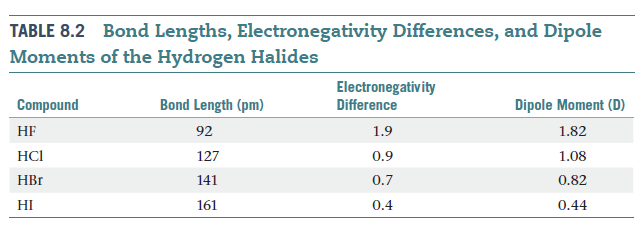Problem: From the data in the following table, calculate the effective charges on the H atom of the HBr molecule in units of the electronic charge, e.

FREE Expert Solution
84% (382 ratings)
FREE Expert Solution

$\overline{){\mathbf{q}}{\mathbf{=}}\frac{\mathbf{\mu }}{\mathbf{r}}}$

84% (382 ratings)
Problem Details

From the data in the following table, calculate the effective charges on the H atom of the HBr molecule in units of the electronic charge, e.